# Detecting blobs and connections using Python: An Image Processing Introduction

Blobs are pixels that are grouped or connected together that share common properties in an image. Blobs may take different kinds of objects In this article, we will attempt to correctly detect these blobs using this image: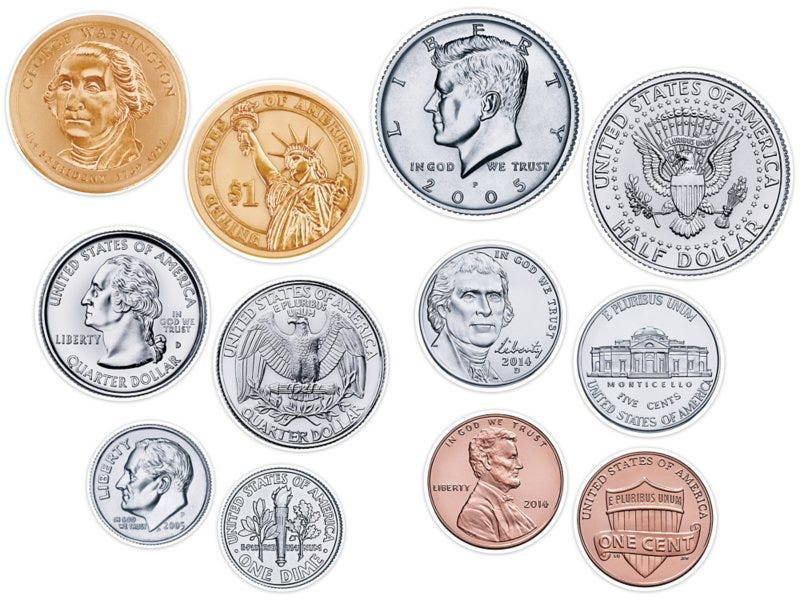A set of US coins

Our aim is to be able to detect the coins correctly. In order to do this we need to transform the image into a binary format since blobs could be identified as bright areas in a dark background or vice-versa.

`from skimage.io import imread, imshowfrom skimage.color import rgb2grayim = rgb2gray(imread(‘coins.jfif’))im_bw = im < 0.85imshow(im_bw)`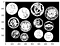Binarized image of coins

Once we have binarized our image, we can now use the three (3) methods available in detecting blobs and these are: 1) Laplacian of Gaussian, 2) Difference of Gaussian, and 3)Determinant of Hessian

`blobs_log = blob_log(im_bw, max_sigma=30, num_sigma=10, threshold=.1)# Compute radii in the 3rd column.blobs_log[:, 2] = blobs_log[:, 2] * sqrt(2)blobs_dog = blob_dog(im_bw, max_sigma=30, threshold=.1)blobs_dog[:, 2] = blobs_dog[:, 2] * sqrt(2)blobs_doh = blob_doh(im_bw, max_sigma=30, threshold=.01)blobs_list = [blobs_log, blobs_dog, blobs_doh]colors = ['blue', 'lime', 'red']titles = ['Laplacian of Gaussian', 'Difference of Gaussian',          'Determinant of Hessian']sequence = zip(blobs_list, colors, titles)fig, axes = plt.subplots(1, 3, figsize=(9, 3), sharex=True, sharey=True)ax = axes.ravel()for idx, (blobs, color, title) in enumerate(sequence):    ax[idx].set_title(title)    ax[idx].imshow(im_bw, interpolation='nearest')    for blob in blobs:        y, x, r = blob        c = plt.Circle((x, y), r, color=color, linewidth=2, fill=False)        ax[idx].add_patch(c)    ax[idx].set_axis_off()plt.tight_layout()plt.show()`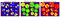Results from different blob detection methods

The results from using the three blob detection methods are quite dirty. However, we could clearly see here that the difference of gaussian has much better result in detecting the coins compared to the other two in this case. You may want to play around with the different parameters for the blob_log function to have better results as well.

Another way of detecting objects in an image beside from blob detection is via connected components. The advantage of this compared to blob detection is that we could detect irregular shaped objects, however we need to use morphological operations (which I discussed in one of my articles) in cleaning our image.

`## Morphing/Cleaning the imagefrom skimage.morphology import erosion, dilation, opening, closingfrom skimage.measure import label, regionpropsfrom skimage.color import label2rgbdef multi_dil(im,num):    for i in range(num):        im = dilation(im)    return imdef multi_ero(im,num):    for i in range(num):        im = erosion(im)    return imim_cleaned = multi_ero(multi_dil(im_bw,5),5)imshow(im_cleaned)`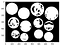Morphed image of coins

As you can see, I dilated the image 5 times and then eroded it 5 times again in order to fill the gaps in the coins. The morphological operations that you will use and the number of times you will apply it may vary depending on the image that you have. After morphing, we can now use the following for identifying the coins:

`## Detecting the coinslabel_im = label(im_cleaned)imshow(label_im)`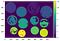Detecting the coins based on their connectivity and color

What I did here is that I used the label function from the skimage library that will label the connected pixels from one another. Each of the coins has their own somewhat a color code value which makes them identifiable from one another. If ever there are coins that are probably overlapping and thus there’s a connection, they might end up with the same color even though they are actually different coins.

And that’s it for blob detection and connected components! Hope I was able to help you regarding this topic.

A developer by day, a programmer by night

## More from Cymon Marcaida

A developer by day, a programmer by night